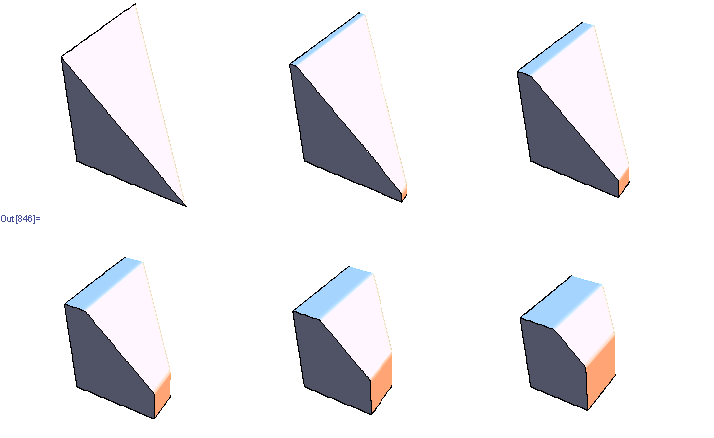## Monday, December 17, 2007

### Sampling doubly stochastic matrices

Stochastic matrices are easy to get -- just normalize the rows. Doubly stochastic matrices require more work -- simply normalizing columns/rows will not converge may take few dozen iterations to converge. One approach that works is to do constrained optimization, finding closest (in least squared sense) doubly stochastic matrix to given matrix. Another approach is to start with a permutation matrix and follow a Markov chain that modifies 4 elements randomly at each step in a way that keeps the matrix doubly stochastic. Here is the implementation of these two methods in Mathematica (notebook in the same directory)

http://www.yaroslavvb.com/research/qr/doubly-stochastic/doubly-stochastic.html

As Jeremy pointed out, Birkhoff's theorem provides necessary and sufficient conditions for a matrix to be doubly stochastic -- it must be a convex combination of permutation matrices. It seems hard to sample from the set of valid convex combinations though. For instance, here's a set of 3 dimensional slices through the set of valid convex combinations for 3x3 matrices (there are 6 permutation matrices, so the space is 5 dimensional).Update: here's the updated notebook with 2 more algorithms, suggested by Dr.Teh and Jeremy below. Turns out that sampling random convex combinations is fairly easy -- almost all the time uniformly sampled convex combination will produce a doubly-stochastic matrix. However, matrices end up looking pretty uniform
http://www.yaroslavvb.com/research/qr/doubly-stochastic/doubly-stochastic3.html

#### 8 comments:

Jeremy said...

Your second approach reminds me of the following theorem (taken from Combinatorial Matrix Theory by Brualdi and Ryser):

A matrix A is doubly stochastic iff there exists permutation matrices P_1, ..., P_t and real numbers c_1, ... , c_t such that

A = c_1 P_1 + ... + c_t P_t

and

c_1 + ... + c_t = 1.

ydub said...

I have a question: why won't iteratively normalizing columns and rows work? Isn't it IPF? cheers, -yw

Yaroslav said...

Jeremy -- thanks for the pointer. I also found it as Thm. 8.7.1 in Horn and Johnson

Dr Teh -- you are right, it's IPF, I made a mistake of running it for too few iterations, if I run it longer it tends to converge (within 10^-10 of doubly stochastic) after 30 iterations for 3x3 matrices, less for larger matrices
http://www.yaroslavvb.com/research/qr/doubly-stochastic/doubly-stochastic2.html

Yaroslav said...

In fact, convergence of IPF to doubly-stochastic matrix was proven back in 1964

Yaroslav said...

(convergence is guaranteed only if all matrix entries are strictly positive)

dfacto said...

Isn't this simpler and equivalent? In Matlab notation: [T,~]=qr(rand(n));T=T.^2;

GG. said...

The article is great
http://wez-pozyczke.pl

draj said...

Excellent machine learning blog,thanks for sharing...
Seo Internship in Bangalore
Smo Internship in Bangalore
Digital Marketing Internship Program in Bangalore